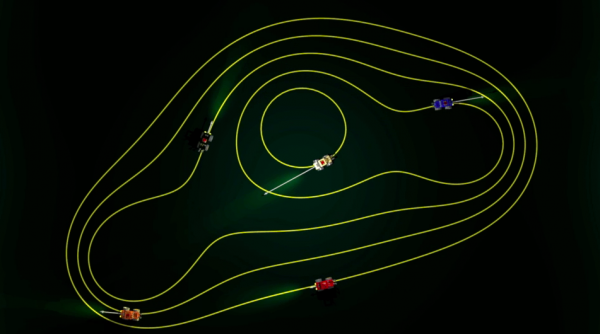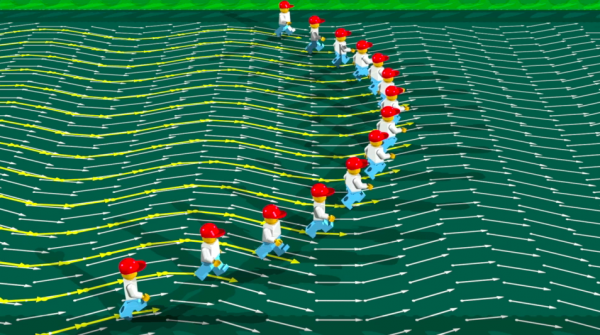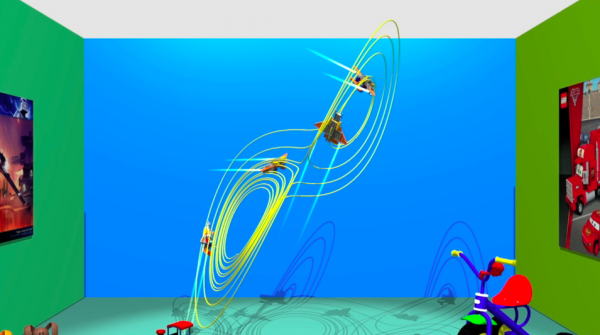# The lego race

At the end of the 17th century, Gottfried Wilhelm Leibniz (1646-1716) and Isaac Newton (1643-1727), independently one from the other, invented a brilliant mathematical tool:  infinitesimal calculus or differential and integral calculus. This is an incredibly efficient crystal ball to predict the future, provided the system in question is governed by a differential equation. This second chapter is about an introduction to the subject in the Lego world.How can we define the speed of a lego man that walks? The average speed is the ratio of the distance travelled, and the time that  it took to do so. With that we can calculate the average speed for each step.But what about a driving car? The idea here is to consider the motion of a car as a succession of small steps, so small that they cannot be noticed. This is the basis for the derivative or differential calculusImagine a flowing river. For each point of the river, it is possible to calculate the speed of the water at that point. We then take a drawing of the river and draw an arrow on the point in question. The length indicates the speed, and the direction indicates the direction of the speed. Such an arrow is called a vector, and we have such a vector for each point of the river. Mathematicians call this a vector field.Integral calculus is the opposite of differential calculus. The task is now to calculate trajectories in a given vector field. The film shows how lego men moving through a vector field have no choice but to follow a predetermined path. This is known mathematically as the Cauchy-Lipschitz theorem and summarises the concept of determinism: with a given vector field, and a given starting position, there is a unique trajectory starting from that point, and this trajectory is tangent everywhere to the velocity vectors.Determinism as we have defined it has its limits, as we can show with a simple example.  In 1879, the physicist James Clerk Maxwell (1831-1879) insisted on the importance of initial conditions for physical phenomena.« There is a maxim…that the same causes will always produce the same effects [...] There is another maxim which must not be confounded with the first, which asserts that “like causes produce like effects. This is only true when small variations  in the initial circumstances produce only small variations in the final state of the system. In a great many physical phenomena this condition is satisfied; but there are other cases in which  a small initial variation may produce a very great change in the final state of the system. »At the end of this chapter we see our lego men flying in their small spacecrafts. The images should convince you that now, in three dimensions, the situation can become very complicated..